Interesting performance comparisons between pandas and numpy

Pandas and Numpy are two packages that are core to a lot of data analysis. In this post I will compare the performance of numpy and pandas.

tl;dr:

• numpy consumes less memory compared to pandas
• numpy generally performs better than pandas for 50K rows or less
• pandas generally performs better than numpy for 500K rows or more
• for 50K to 500K rows, it is a toss up between pandas and numpy depending on the kind of operation
In :
import pandas as pd
import matplotlib.pyplot as plt
plt.style.use("seaborn-pastel")
%matplotlib inline
import seaborn.apionly as sns
import numpy as np
from timeit import timeit
import sys

In :
iris = sns.load_dataset('iris')

In :
data = pd.concat([iris]*100000)
data_rec = data.to_records()

In :
print (len(data), len(data_rec))

15000000 15000000


Here I have loaded the iris dataset and replicated it so as to have 15MM rows of data. The space requirement for 15MM rows of data in a pandas dataframe is more than twice that of a numpy recarray.

In :
MB = 1024*1024
print("Pandas %d MB " % (sys.getsizeof(data)/MB))
print("Numpy %d MB " % (sys.getsizeof(data_rec)/MB))

Pandas 1506 MB
Numpy 686 MB


A snippet of the data shown below.

In :
data.head()

Out:
sepal_length sepal_width petal_length petal_width species
0 5.1 3.5 1.4 0.2 setosa
1 4.9 3.0 1.4 0.2 setosa
2 4.7 3.2 1.3 0.2 setosa
3 4.6 3.1 1.5 0.2 setosa
4 5.0 3.6 1.4 0.2 setosa
In :
Expand Code

In this post, performance metrics for a few different categories are compared between numpy and pandas:

• operations on a column of data, such as mean or applying a vectorised function
• operations on a filtered column of data
• vector operations on a column or filtered column

## Operations on a Column¶

Here some performance metrics with operations on one column of data. The operations involved in here include fetching a view, and a reduction operation such as mean, vectorised log or a string based unique operation. All these are O(n) calculations. The mean calculation is orders of magnitude faster in numpy compared to pandas for array sizes of 100K or less. For sizes larger than 100K pandas maintains a lead over numpy.

In :
bench(data, "data.loc[:, 'sepal_length'].mean()",
data_rec, "np.mean(data_rec.sepal_length)",
title="Mean on Unfiltered Column")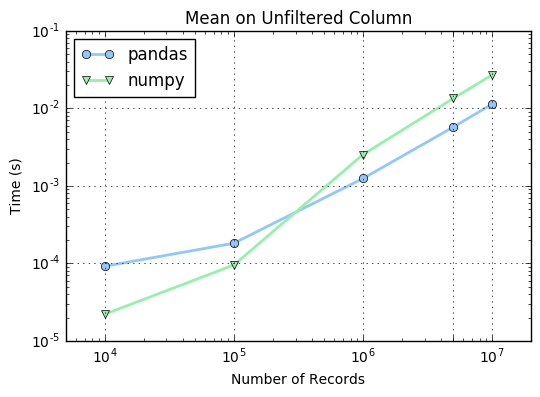Below, the vectorized log operation is faster in numpy for sizes less than 100K but pandas costs about the same for sizes larger than 100K.

In :
bench(data, "np.log(data.loc[:, 'sepal_length'])",
data_rec, "np.log(data_rec.sepal_length)",
title="Vectorised log on Unfiltered Column")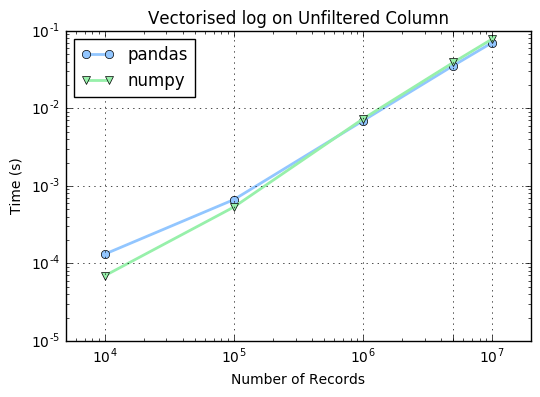The one differentiating aspect about the test below is that the column species is of string type. The operation demonstrated is a unique calculation. We observe that the unique calculation is roughly an order of magnitude faster in pandas for sizes larger than 1K rows.

In :
bench(data, "data.loc[:,'species'].unique()",
data_rec, "np.unique(data_rec.species)",
grid=np.array([100, 1000, 10000, 100000, 1000000]),
title="Unique on Unfiltered String Column")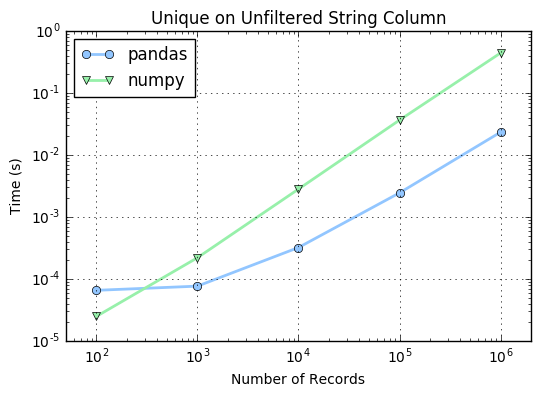## Operations on a Filtered Column¶

Below we perform the same tests as above, except that the column is not a full view, but is instead a filtered view. The filters are simple filters with an arithmetic bool comparison for the first two and a string comparison for the third below.

Below, mean is calculated for a filtered column sepal_length. Here performance of pandas is better for row sizes larger than 10K. In the mean on unfiltered column shown above, pandas performed better for 1MM or more. Just having selection operations has shifted performance chart in favor of pandas for even smaller number of records.

In :
bench(data, "data.loc[(data.sepal_width>3) & \
(data.petal_length<1.5), 'sepal_length'].mean()",
data_rec, "np.mean(data_rec[(data_rec.sepal_width>3) & \
(data_rec.petal_length<1.5)].sepal_length)",
grid=np.array([1000, 10000, 100000, 1000000]),
title="Mean on Filtered Column")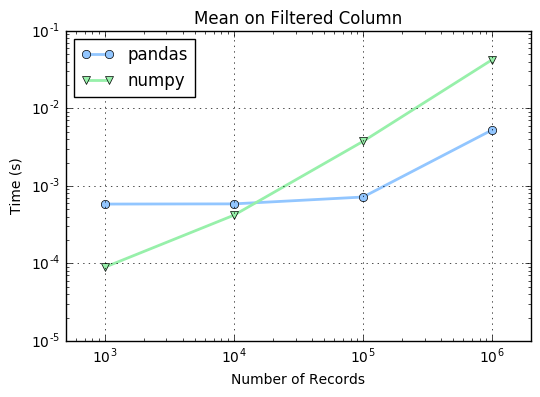For vectorised log operation on a unfiltered column shown above, numpy performed better than pandas for number of records less than 100K while the performance was comparable for the two for sizes larger than 100K. But the moment you introduce a filter on a column, pandas starts to show an edge over numpy for number of records larger than 10K.

In :
bench(data, "np.log(data.loc[(data.sepal_width>3) & \
(data.petal_length<1.5), 'sepal_length'])",
data_rec, "np.log(data_rec[(data_rec.sepal_width>3) & \
(data_rec.petal_length<1.5)].sepal_length)",
grid=np.array([1000, 10000, 100000, 1000000]),
title="Vectorised log on Filtered Column")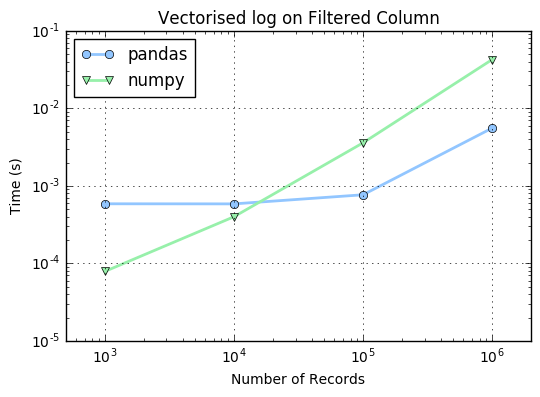Here is another example of a mean reduction on a column but with a string filter. We see a similar behavior where numpy performs significantly better at small sizes and pandas takes a gentle lead for larger number of records.

In :
bench(data, "data[data.species=='setosa'].sepal_length.mean()",
data_rec, "np.mean(data_rec[data_rec.species=='setosa'].sepal_length)",
grid=np.array([1000, 10000, 100000, 1000000]),
title="Mean on (String) Filtered Column")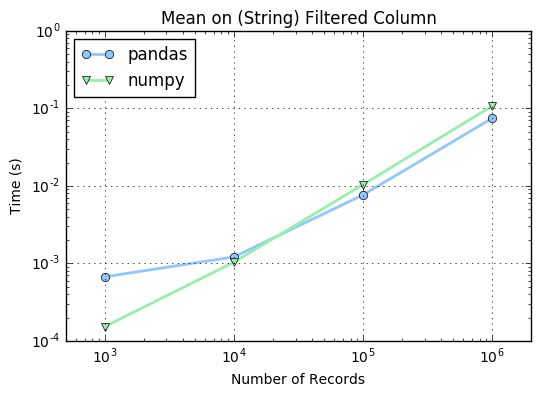## Vectorized Operation on a Column¶

In this last section, we do vectorised arithmetic using multiple columns. This involves creating a view and vectorised math on these views. Even when there is no filter, pandas has a slight edge over numpy for large number of records. For smaller than 100K records, numpy performs significantly better.

In :
bench(data, "data.petal_length * data.sepal_length + \
data.petal_width * data.sepal_width",
data_rec, "data_rec.petal_length*data_rec.sepal_length + \
data_rec.petal_width * data_rec.sepal_width",
title="Vectorised Math on Unfiltered Columns")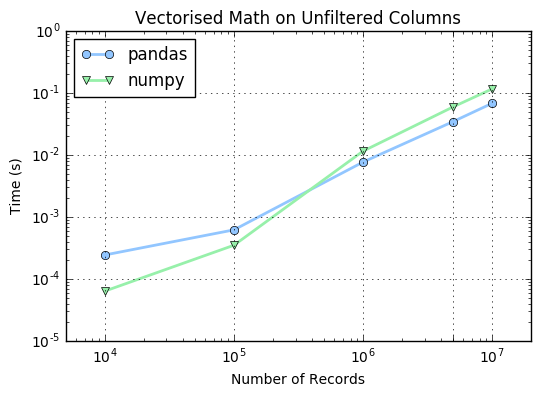In the following figure, the filter involves vectorised arithmetic operation, and mean reduction is computed on the filtered column. The presence of a filter makes pandas significantly faster for sizes larger than 100K, while numpy maitains a lead for smaller than 10K number of records.

In :
bench(data, "data.loc[data.sepal_width * data.petal_length > \
data.sepal_length, 'sepal_length'].mean()",
data_rec, "np.mean(data_rec[data_rec.sepal_width * data_rec.petal_length \
> data_rec.sepal_length].sepal_length)",
title="Vectorised Math in Filtering Columns",
grid=np.array([100, 1000, 10000, 100000, 1000000]))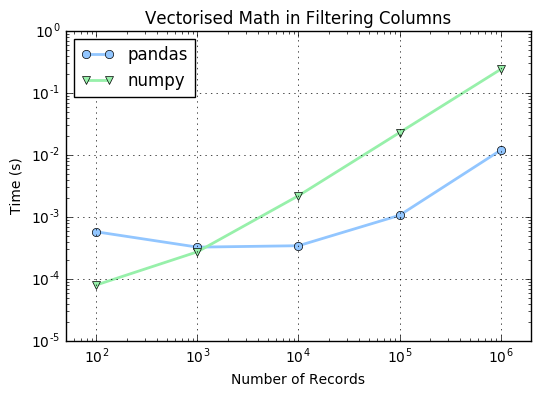## Conclusion¶

Pandas is often used in an interactive environment such as through Jupyter notebooks. In such a case, any performance loss from pandas will be in significant. But if you have smaller pandas dataframes (<50K number of records) in a production environment, then it is worth considering numpy recarrays.

• numpy consumes (roughtly 1/3) less memory compared to pandas
• numpy generally performs better than pandas for 50K rows or less
• pandas generally performs better than numpy for 500K rows or more
• for 50K to 500K rows, it is a toss up between pandas and numpy depending on the kind of operation

python   programming   development   pandas   numpy

Related PostI am Goutham Balaraman, and I explore topics in quantitative finance, programming, and data science. You can follow me @gsbalaraman.

Checkout my bookUpdated posts from this blog and transcripts of Luigi's screencasts on YouTube is compiled into QuantLib Python Cookbook .